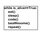# The most weird theorem of all time?

If a class has 23 students in it then the probability that at least two of the students share a birthday is about 0.5. Surprised? If there are 50 students in a class then it's virtually certain that two will share the same birthday. This seems to go against common sense but is absolutely correct. Let's look at the probabilities a step at a time.

For one person, there are 365 distinct birthdays. For two people, there are 364 different ways that the second could have a birthday without matching the first. If there is no match after two people, the third person has 363 different birthdays that do not match the other two. So, the probability of a match is 1 - (365)(364)(363)/(365)(365)(365). This leads to the following formula for calculating the probability of a match with N birthdays is 1 - (365)(364)(363)...(365 - N + 1)/(365)^N. Running this through a computer gives that a probability of over .5 is obtained after 23 dates! If you like please reshare as many times as possible!!Note by Harsh Shrivastava
7 years, 3 months ago

This discussion board is a place to discuss our Daily Challenges and the math and science related to those challenges. Explanations are more than just a solution — they should explain the steps and thinking strategies that you used to obtain the solution. Comments should further the discussion of math and science.

When posting on Brilliant:

• Use the emojis to react to an explanation, whether you're congratulating a job well done , or just really confused .
• Ask specific questions about the challenge or the steps in somebody's explanation. Well-posed questions can add a lot to the discussion, but posting "I don't understand!" doesn't help anyone.
• Try to contribute something new to the discussion, whether it is an extension, generalization or other idea related to the challenge.
• Stay on topic — we're all here to learn more about math and science, not to hear about your favorite get-rich-quick scheme or current world events.

MarkdownAppears as
*italics* or _italics_ italics
**bold** or __bold__ bold
- bulleted- list
• bulleted
• list
1. numbered2. list
1. numbered
2. list
Note: you must add a full line of space before and after lists for them to show up correctly
paragraph 1paragraph 2

paragraph 1

paragraph 2

[example link](https://brilliant.org)example link
> This is a quote
This is a quote
    # I indented these lines
# 4 spaces, and now they show
# up as a code block.

print "hello world"
# I indented these lines
# 4 spaces, and now they show
# up as a code block.

print "hello world"
MathAppears as
Remember to wrap math in $$ ... $$ or $ ... $ to ensure proper formatting.
2 \times 3 $2 \times 3$
2^{34} $2^{34}$
a_{i-1} $a_{i-1}$
\frac{2}{3} $\frac{2}{3}$
\sqrt{2} $\sqrt{2}$
\sum_{i=1}^3 $\sum_{i=1}^3$
\sin \theta $\sin \theta$
\boxed{123} $\boxed{123}$

## Comments

Sort by:

Top Newest

It's the Birthday Problem!

- 7 years, 3 months ago

Log in to reply

thought that I would get atleast 3likes & 5 comments,but not a single!!;

- 7 years, 3 months ago

Log in to reply

×

Problem Loading...

Note Loading...

Set Loading...